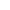Principle Foundations      Home PageProblems in Regression Analysis  and their CorrectionsMulticollinearity refers to the case in which two or more explanatory variables in the regression model are highly correlated, making it difficult or impossible to isolate their individual effects on the dependent variable. With multicollinearity, the estimated OLS coefficients may be statistically insignificant (and even have the wrong sign) even though R2 may be "high". Multicollinearity can some times be overcome or reduced by collecting more data, by utilizing a priory information, by transforming the functional relationship, or by dropping one of the higly collinear variables. Two or more independent variables are perfectly collinear if one or more of the variables can be expressed as a linear combination of the other variable(s). For example, there is perfect multicollinearity between X1 and X2 if X1 = 2X2 or. If two or more explanatory variables are perfectly linearly correlated, it will be impossible to calculate OLS estimates of the parameters because the system of normal equations will contain two or more equations that are not independent. High, but not perfect, multicollinearity refers to the case in which two or more independent variables in the regression model are highly correlated. This may make it difficult or impossible to isolate the effect that each of the highly collinear explanatory variables has on the dependent variable. However, The OLS estimated coefficients are still unbiased (if the model is properly specified). Furthermore, if the principal aim is prediction, multicollinearity is not a problem if the same multicollinearity pattern persists during the forecasted period.   The classic case of multicollinearity occurs when none of explanatory variables in the OLS regression is statistically significant (an some may even have the wrong sign), even though R2 may be high (say, between 0.7 and 1.0). In the less clear-cut cases, detecting multicollinearity may be more difficult. High, simple, or partial correlation coefficients amongst explanatory variables are sometimes used as a measure of multicollinearity. However, serious multicollinearity can be present even if simple or partial correlation coefficients are relatively low (ie less than 0.5).   Serious multicollinearity may sometimes be corrected by 1) extending the size of the sample data, 2) utilizing a priory information (for example, we may know from a previous study that b2=0.25b1), 3) transforming the functional relationship, or 4) dropping one of the highly collinear variables (however, this may lead to specification bias or error if theory tells us that the dropped variable should be included in the model).     HETEROSKEDASTICITY      If the OLS assumption that the variance of the error term is constant for all values of the independent variables does not hold, we face the problem of heteroskedasticity. This leads to unbiased but inefficient (ie, larger than minimum variance) estimates of the standard errors (and thus, incorrect statistical tests confidence intervals). One test for heteroskedasticity involves arranging the data from small to large values of the independent variable, X, and running two regressions, one for small values of X and one for large values, omitting, say, one-fifth of the middle observations. Then, we test that the ratio of the error sum of squares (ESS) of the second to the first regression is significantly different from zero, using the F table with (n-d-2k)/2 d.f, where n is the total number of observations, d is the numbers of omitted observations and K is the number of estimated parameters. If the error variance is proportional to X2 (often the case), heteroskedasticity can be overcome by dividing every term of the model by X and then reestimating the regression using the transformed variables. Heteroskedasticity refers to the case in which the variance of the error term is not constant for all values of the independent variable. That is,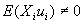, so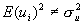. This violates the third assumption of the OLS regression model. it occurs primarily in cross sectional data. For example, the error variance associated with the expenditures of low-income families is usually smaller than for high-income families because most of the expenditures of low-income families are on necessities, with little room for discretion.   Figure 9-1a shows homoskedastic (i.e., constant variance) disturbances, while Figure 9-1b, c, and d shows heteroskedastic disturbances. In Figure 9-1b,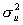increases with Xi. In Figure 9-1c,decreases with Χi. In Figure 9-1d,first decreases and then increases as Xi increases. In economics, the heteroskedasticity shown in Figure 9-1b is the most common, so the discussion that follows refers to that.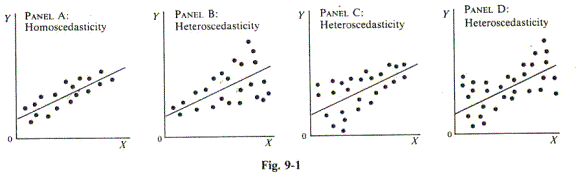With heteroskedasticity, the OLS parameter estimate are still unbiased and consistent, but they are inefficient (i.e., they have larger than minimum variances). Furthermore, the estimated variances of the parameters are biased, leading to incorrect statistical tests for the parameters and biased confidence intervals. The presence of heteroskedasticity can be tested by arranging the data from small to large values of the independent variable, Xi, and then running two separate regressions, one for small values of Xi and one for large values of Xi, omitting some (say, one-fifth) of the middle observations. Then the ratio of the error sum of squares of the second regression to the error sum of squares of the first regression (that is, ESS2/ESS1) is tested to see if it is significantly different from zero. The F distribution is used for this test with (n - d -2k)/2 degrees of freedom, where n is the total number of observations, d is the number of omitted observations, and k is the number of estimated parameters. This is the Goldfeld-Quandt test for heteroskedasticity and is most appropriate for large samples (i.e., for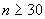). If no middle observations are omitted, the test is still correct, but it will have a reduced power to detect heteroskedasticity.     AUTOCORRELATION     When the error term in one time period is positively correlated with the error term in the previous time period, we face the problem of (positive first-order) autocorrelation. This is common in time-series analysis and leads to downward-biased standard errors (and, thus, to incorrect statistical tests and confidence intervals). The presence of first-order auto relation is tested by utilizing the table of the Durbin-Watson statistic (App.8) at the 5 or 1% levels of significance for n observations and k' explanatory variables. If the calculated value of d from Eq.(9.1) is smaller than the tabular value of dL (lower limit), the hypothesis of positive first-order autocorrelation is accepted.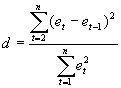the hypothesis is rejected if d>dU (upper limit), and the test is inconclusive if dL